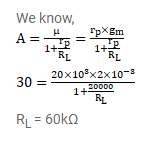# A triode has mutual conductance=2.0 millimho and

Question:

A triode has mutual conductance $=2.0$ millimho and plate resistance $=20 \mathrm{k} \Omega$. It is desired to amplify a signal by a factor of 30 . What load resistance should be added in the circuit?

Solution: